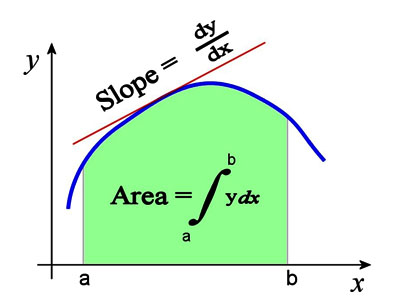ΓραμματείαSecretariat: 2410 684574 | ΦοιτητικάStudents: 2410 684387 g-ds@uth.gr
Select Page

# Mathematical Analysis

Y101

1

#### Hours/Week - ECTS

5 – 5### Learning Outcomes

Beyond rules and theorems, the course seeks to show the mathematical way of thinking, to develop from the student’s combinatorial ability and ability to solve problems.

Objective goals:

• sufficiency of knowledge to follow the technological courses taught
• critical skill and intellectual discipline
• understanding the fundamental concepts of functions of one and many variables
• knowledge of the mathematical “language” with which the student structures solidly and rigorously the concepts, phenomena and laws of science and technology

### Indicative Module Content

• Sets. The concept of visualization. Real numbers. Powers of R. Real numbers. The extended set R. Intervals. Distance. Point area. Classification of points of R. Open and closed sets.
• Sequences of real numbers. Sequence limit. Acts with limits. Cauchy criterion. Monotonous sequences. Systolic sequence. Recursive sequences. Difference equations.
• Series of real functions. Basic criteria of series convergence.
• Function continuation. Basic theorems.
• Derivative of a function. Basic theorems. Leibniz rule.
• Inverses of trigonometric functions. Hyperbolic functions and their inverses.
• Differential. Higher order derivatives and differentials.
• Approximation of functions with polynomials. Taylor (Maclaurin) polynomial. Powertrains.
• Indefinite integral. Integration methods. Riemann integral. Fundamental theorems. Average value theorem of Integral Calculus. Production of integrals.
• Generalized integral. Basic convergence propositions.
• Applications of Definite Integral. Fourier series.
• Ordinary differential equations. Equations of separable variables. Homogeneous. First order linear equations. Second order linear equations with constant coefficients. Euler equation.
• Euclidean space Rⁿ. Point area. Classification of points of Rⁿ. Open and closed sets.
• Sequences. Basic theorems.
• Functions of several variables. Limit of a function. Boundary properties. Function continuation. Properties of continuous functions.
• Partial derivatives of first and higher order. Differentiable function. Total differential. Derivative of complex function of first and higher order. Higher class differential. Determinant derivative. Functional determinants. Complex functions. Generalization. System reversal. Laplace transforms equations. Derivative in direction. Average value theorem. Taylor (Maclaurin) type. Local and committed extremes.
• Double and triple integrals.
• Curved integrals of the first and second kind.
• Fields. Slope. Deviation. Rotation. Rise up. Green’s theorem. Defining the vector function.